# Data Structures in Python

## Data Structures in Python with Examples

In this article, I am going to discuss Data Structures in Python with Examples. Please read our previous article where we gave a brief introduction to Python Programming for Data Science. At the end of this article, you will understand the following pointers.

1. Lists
2. Tuples
3. Indexing and Slicing
4. Iterating through a Sequence
5. Functions for all Sequences
6. Using Enumerate()
7. Operators and Keywords for Sequences
8. The xrange() function
9. List Comprehensions
10. Generator Expressions
11. Dictionaries and Sets
##### Python Data Structures:

In python, there are quite a few data structures available. They are used to store a group of individual objects as a single entity.

##### Sequences

Sequences are those, which contain a group of elements, whose purpose is to store and process a group of elements. In python, strings, lists, tuples, and dictionaries are very important sequence data types.

##### Lists in Python

Python provides a number of compound data types, which are commonly referred to as sequences. The list data type is one of the most commonly used and versatile data types in Python. A list is created in Python programming by putting all of the items (elements) inside square brackets [], separated by commas. It can contain an unlimited number of items of various types (integer, float, string etc.).

```# empty list
my_list = []

# list of integers
my_list = [1, 2, 3]

# list with mixed data types
my_list = [1, "Hello", 3.4]
```

Another list can be included as an item in a list. This is referred to as a nested list.

```# nested list
my_list = ["mouse", [8, 4, 6], ['a']]
```
##### Access of List Elements in Python

The elements of a list can be accessed in a variety of ways.

##### Index of Lists in Python

To access a list item, we can use the index operator []. In Python, indices begin at zero. As a result, a list with 5 elements will have an index ranging from 0 to 4. Attempting to access indexes other than those listed above will result in an IndexError. The index must be a positive integer. We can’t use floats or other types because it will cause a TypeError. Nested indexing is used to access nested lists.

```# List indexing

my_list = ['p', 'r', 'o', 'b', 'e']

# Output: p
print(my_list)

# Output: o
print(my_list)

# Output: e
print(my_list)

# Nested List
n_list = ["Happy", [2, 0, 1, 5]]

# Nested indexing
print(n_list)

print(n_list)

# Error! Only integer can be used for indexing
print(my_list[4.0])
```
###### Output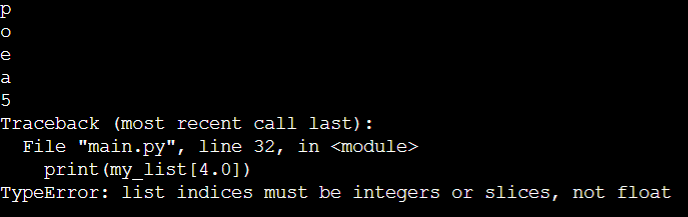Python sequences support negative indexing. The index of -1 denotes the last item, the index of -2 the second last item, and so on.

```# Negative indexing in lists
my_list = ['p','r','o','b','e']

print(my_list[-1])

print(my_list[-5])
```

When we run the preceding program, we will get the following results: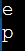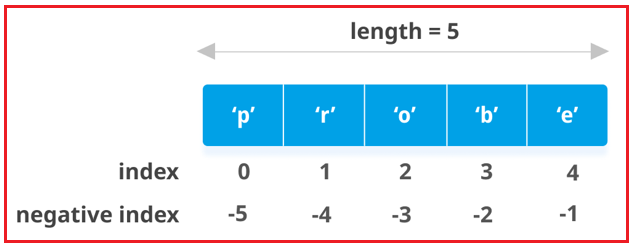##### In Python, how do you slice lists?

Using the slicing operator, we can access a subset of items in a list using ‘:’ (colon).

```# List slicing in Python

my_list = ['p','r','o','g','r','a','m','i','z']

# elements 3rd to 5th
print(my_list[2:5])

# elements beginning to 4th
print(my_list[:-5])

# elements 6th to end
print(my_list[5:])

# elements beginning to end
print(my_list[:])
```
###### Output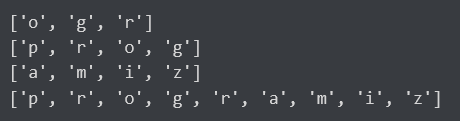Slicing is best visualized by placing the index between the elements, as shown below. So, in order to access a range, we’ll need two indices that will slice that portion of the list. Lists, unlike strings and tuples, are mutable, which means that their elements can be changed. To change an item or a range of items, we can use the assignment operator =.

```# Correcting mistake values in a list
odd = [2, 4, 6, 8]

# change the 1st item
odd = 1

print(odd)

# change 2nd to 4th items
odd[1:4] = [3, 5, 7]

print(odd)
```
###### Output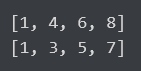We can add a single item to a list by using the append() method, or we can add multiple items by using the extend() method.

```# Appending and Extending lists in Python
odd = [1, 3, 5]

odd.append(7)

print(odd)

odd.extend([9, 11, 13])

print(odd)
```
###### Output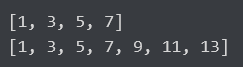To combine two lists, we can also use the + operator. This is also known as concatenation. The * operator iterates over a list for the specified number of times.

```# Concatenating and repeating lists
odd = [1, 3, 5]

print(odd + [9, 7, 5])

print(["re"] * 3)
```
###### Output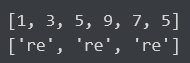Furthermore, we can insert a single item at a specific location by using the insert() method, or we can insert multiple items by squeezing them into an empty slice of a list.

```# Demonstration of list insert() method
odd = [1, 9]
odd.insert(1,3)

print(odd)

odd[2:2] = [5, 7]

print(odd)
```
###### Output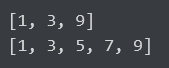##### Delete/Remove List Elements in Python

Using the keyword del, we can remove one or more items from a list. It has the ability to completely delete the list.

```# Deleting list items
my_list = ['p', 'r', 'o', 'b', 'l', 'e', 'm']

# delete one item
del my_list

print(my_list)

# delete multiple items
del my_list[1:5]

print(my_list)

# delete entire list
del my_list

# Error: List not defined
print(my_list)
```
###### Output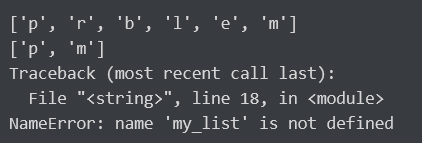To remove the given item, we can use the remove() method, or we can use the pop() method to remove an item at the given index. If no index is provided, the pop() method removes and returns the last item. This assists us in implementing lists as stacks (first in, last out data structure). To empty a list, we can also use the clear() method.

```my_list = ['p','r','o','b','l','e','m']
my_list.remove('p')

# Output: ['r', 'o', 'b', 'l', 'e', 'm']
print(my_list)

# Output: 'o'
print(my_list.pop(1))

# Output: ['r', 'b', 'l', 'e', 'm']
print(my_list)

# Output: 'm'
print(my_list.pop())

# Output: ['r', 'b', 'l', 'e']
print(my_list)

my_list.clear()

# Output: []
print(my_list)
```
###### Output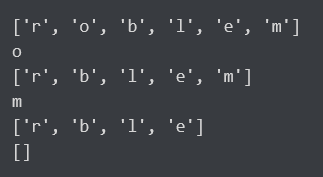Finally, by assigning an empty list to a slice of elements, we can delete items from a list.

```>>> my_list = ['p','r','o','b','l','e','m']
>>> my_list[2:3] = []
>>> my_list
['p', 'r', 'b', 'l', 'e', 'm']
>>> my_list[2:5] = []
>>> my_list
['p', 'r', 'm']
```

#### Tuples in Python

In Python, a tuple is similar to a list. The difference is that we cannot change the elements of a tuple once it has been assigned, whereas we can change the elements of a list.

##### Creating a Tuple

A tuple is formed by putting all of the items (elements) inside parentheses () and separating them with commas. The parentheses are optional, but it is recommended that they be used. A tuple can contain any number of items of various types (integer, float, list, string, etc.).

```# Different types of tuples

# Empty tuple
my_tuple = ()
print(my_tuple)

# Tuple having integers
my_tuple = (1, 2, 3)
print(my_tuple)

# tuple with mixed datatypes
my_tuple = (1, "Hello", 3.4)
print(my_tuple)

# nested tuple
my_tuple = ("mouse", [8, 4, 6], (1, 2, 3))
print(my_tuple)
```
###### Output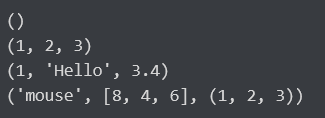A tuple can also be formed without the use of parentheses. This is referred to as tuple packing.

```my_tuple = 3, 4.6, "dog"
print(my_tuple)

# tuple unpacking is also possible
a, b, c = my_tuple

print(a)      # 3
print(b)      # 4.6
print(c)      # dog
```
###### OutputCreating a tuple with only one element is difficult. Having just one element within parentheses is insufficient. A trailing comma will be required to indicate that it is, in fact, a tuple.

```my_tuple = ("hello")
print(type(my_tuple))  # <class 'str'>

# Creating a tuple having one element
my_tuple = ("hello",)
print(type(my_tuple))  # <class 'tuple'>

# Parentheses is optional
my_tuple = "hello",
print(type(my_tuple))  # <class 'tuple'>
```
###### Output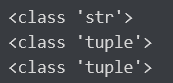#### Accessing Elements of a Tuple in Python

The elements of a tuple can be accessed in a variety of ways.

##### 1. Indexation

To access an item in a tuple, we can use the index operator [], where the index starts at 0. As a result, a tuple with six elements will have indices ranging from 0 to 5. Attempting to access an index that is not within the tuple index range (6, 7 in this example) will result in an IndexError.

We can’t use floats or other types because the index must be an integer. This will produce a TypeError. As shown in the example below, nested tuples are accessed using nested indexing.

```# Accessing tuple elements using indexing
my_tuple = ('p','e','r','m','i','t')

print(my_tuple)   # 'p'
print(my_tuple)   # 't'

# IndexError: list index out of range
# print(my_tuple)

# Index must be an integer
# TypeError: list indices must be integers, not float
# my_tuple[2.0]

# nested tuple
n_tuple = ("mouse", [8, 4, 6], (1, 2, 3))

# nested index
print(n_tuple)       # 's'
print(n_tuple)       # 4
```
###### Output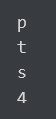##### 2. Negative Indexing in Python

Python sequences support negative indexing. The index of -1 denotes the last item, the index of -2 the second last item, and so on.

```# Negative indexing for accessing tuple elements
my_tuple = ('p', 'e', 'r', 'm', 'i', 't')

# Output: 't'
print(my_tuple[-1])

# Output: 'p'
print(my_tuple[-6])
```
###### Output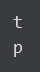Using the slicing operator colon :, we can access a range of items in a tuple.

```# Accessing tuple elements using slicing
my_tuple = ('p','r','o','g','r','a','m','i','z')

# elements 2nd to 4th
# Output: ('r', 'o', 'g')
print(my_tuple[1:4])

# elements beginning to 2nd
# Output: ('p', 'r')
print(my_tuple[:-7])

# elements 8th to end
# Output: ('i', 'z')
print(my_tuple[7:])

# elements beginning to end
# Output: ('p', 'r', 'o', 'g', 'r', 'a', 'm', 'i', 'z')
print(my_tuple[:])
```
###### Output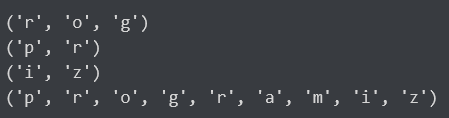Slicing is best visualized by placing the index between the elements. So, if we want to access a range, we need the index that will slice the tuple portion.

##### 3. Changing a Tuple in Python

Tuples, unlike lists, are immutable. This means that once a tuple’s elements have been assigned, they cannot be changed. However, if the element is a mutable data type, such as a list, its nested items can be changed. We can also assign a tuple to various values (reassignment).

```# Changing tuple values
my_tuple = (4, 2, 3, [6, 5])

# TypeError: 'tuple' object does not support item assignment
# my_tuple = 9

# However, item of mutable element can be changed
my_tuple = 9    # Output: (4, 2, 3, [9, 5])
print(my_tuple)

# Tuples can be reassigned
my_tuple = ('p', 'r', 'o', 'g', 'r', 'a', 'm', 'i', 'z')

# Output: ('p', 'r', 'o', 'g', 'r', 'a', 'm', 'i', 'z')
print(my_tuple)
```
###### Output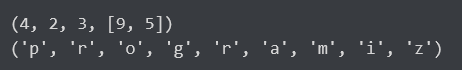To combine two tuples, we can use the + operator. This is known as concatenation. Using the * operator, we can also repeat the elements in a tuple a specified number of times. Both the + and * operations produce a new tuple.

```# Concatenation
# Output: (1, 2, 3, 4, 5, 6)
print((1, 2, 3) + (4, 5, 6))

# Repeat
# Output: ('Repeat', 'Repeat', 'Repeat')
print(("Repeat",) * 3)
```
###### Output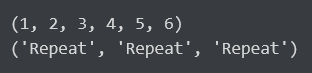##### 4. Deleting a Tuple in Python

As previously stated, we cannot change the elements of a tuple. We cannot delete or remove items from a tuple as a result. However, the keyword del can be used to completely delete a tuple.

```# Deleting tuples
my_tuple = ('p', 'r', 'o', 'g', 'r', 'a', 'm', 'i', 'z')

# can't delete items
# TypeError: 'tuple' object doesn't support item deletion
# del my_tuple

# Can delete an entire tuple
del my_tuple

# NameError: name 'my_tuple' is not defined
print(my_tuple)
```
###### Output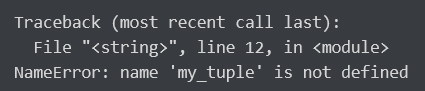Tuples and lists are used in similar situations because they are so similar. However, there are some advantages to using a tuple instead of a list. Some of the main benefits are as follows:

1. Tuples are typically used for heterogeneous (different) data types, while lists are typically used for homogeneous (similar) data types.
2. Because tuples are immutable, iterating through them is faster than iterating through a list. As a result, there is a slight performance boost.
3. Tuples with immutable elements can be used as a dictionary key. This is not possible with lists.
4. If you have non-changing data, implementing it as a tuple ensures that it remains write-protected.
##### Using Enumerate() function in Python

When working with iterators, we frequently encounter the need to keep track of the number of iterations. Python makes it easier for programmers by providing a built-in function enumerate() for this purpose. Enumerate () adds a counter to an iterable and returns it as an enumerate object. This enumerate object can then be used in for loops directly or converted into a list of tuples using the list() method.

```# enumerate function
l1 = ["eat","sleep","repeat"]
s1 = "data"

# creating enumerate objects
obj1 = enumerate(l1)
obj2 = enumerate(s1)

print ("Return type:",type(obj1))
print (list(enumerate(l1)))

# changing start index to 2 from 0
print (list(enumerate(s1,2)))
```
###### Output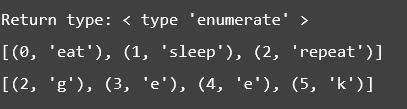##### Xrange Function in Python

The general syntax for defining the xrange is:

xrange(start,end,step)

This defines a range of numbers from start(inclusive) to end(exclusive). There are three parameters that can be passed to the range function:

1. Start: Indicate where the sequence of numbers should begin.
2. End: Indicate where the number sequence should end.

The difference between each number in the sequence is the first step. The end position must be defined. The start and step, on the other hand, are optional. The start and step values are both set to 0 by default.

```print("\n\nSpecify both start,end position and step")
start=2
end=5
step=2
print("start position:",start)
print("end position:",end)
print("step:",step)
z = xrange(3,10,2)#create a sequence of numbers from 3 to 10 with increment of 2
print(z)
for n2 in z:#print the elements using a for loop
print(n2)
```
##### Python List Comprehensions

Python is well-known for encouraging developers and programmers to write code that is efficient, simple to understand, and almost as simple to read. The python list and list compression feature, which can be used within a single line of code to construct powerful functionality, are two of the language’s most distinguishing features.

List comprehensions are used to generate new lists from other iterables such as tuples, strings, arrays, lists, and so on. A list comprehension is made up of brackets that contain the expression that is executed for each element, as well as the for loop that iterates over each element.

1. Loops are less efficient in terms of both time and space.
2. Reduce the number of lines of code required.
3. Iterative statements are converted into formulas.
##### Generator Expressions in Python

To create iterators in Python, we can use both regular functions and generators. Generators are written in the same way as regular functions, but we use yield() instead of return() to return a result. It is more effective as an iterator implementation tool. It is simpler and more convenient to implement because it provides element evaluation on demand. Unlike regular functions, which terminate when they encounter a return statement, generators use a yield statement, which saves the state of the function from the previous call and can be picked up or resumed the next time we call a generator function. Another significant advantage of the generator over a list is that it consumes significantly less memory.

```# Python code to illustrate generator, yield() and next().
def generator():
t = 1
print ('First result is ',t)
yield t

t += 1
print ('Second result is ',t)
yield t

t += 1
print('Third result is ',t)
yield t

call = generator()
next(call)
next(call)
next(call)
```
###### Output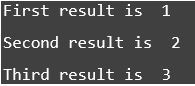The distinction between the Generator function and the Normal function –

1. When the function yields, it is paused and control is transferred to the caller.
2. When the function exits, StopIteration is automatically raised on subsequent calls.
3. Between calls, local variables and their states are remembered.
4. Instead of a return statement, the generator function contains one or more yield statements.
5. Because methods like _next_() and _iter_() are implemented automatically, we can use next to iterate through the items ().

Other expressions can be coded similarly to list comprehensions, but instead of brackets, we use parenthesis. These expressions are intended for situations in which the generator is used immediately by the enclosing function. A generator expression can be used to create a generator without using the yield keyword.

#### Dictionary in Python

A dictionary in Python is an unordered collection of items. A dictionary item has a key/value pair. When the key is known, dictionaries are optimized to retrieve values. Making a dictionary is as simple as putting items inside curly braces and separating them with commas.

An item has a key and a value that is expressed as a pair (key: value). While values can be of any data type and can be repeated, keys must be immutable (string, number, or tuple with immutable elements) and unique.

```# empty dictionary
my_dict = {}

# dictionary with integer keys
my_dict = {1: 'apple', 2: 'ball'}

# dictionary with mixed keys
my_dict = {'name': 'John', 1: [2, 4, 3]}

# using dict()
my_dict = dict({1:'apple', 2:'ball'})

# from sequence having each item as a pair
my_dict = dict([(1,'apple'), (2,'ball')])
```
##### Accessing Dictionary Elements in Python

While other data types use indexing to access values, a dictionary uses keys. Keys can be used with either square brackets [] or the get() method. If we use square brackets [], we get a KeyError if a key is not found in the dictionary. The get() method, on the other hand, returns None if the key is not found.

```# get vs [] for retrieving elements
my_dict = {'name': 'Jack', 'age': 26}

# Output: Jack
print(my_dict['name'])

# Output: 26
print(my_dict.get('age'))

# Trying to access keys which doesn't exist throws error
# Output None

# KeyError
```
###### Output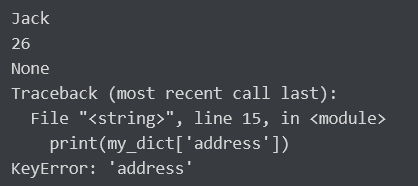##### Changing and Adding Dictionary Elements in Python

Dictionaries are subject to change. Using an assignment operator, we can add new items or change the value of existing items. If the key already exists, the existing value is updated. In the absence of the key, a new (key: value) pair is added to the dictionary.

```# Changing and adding Dictionary Elements
my_dict = {'name': 'Jack', 'age': 26}

# update value
my_dict['age'] = 27

#Output: {'age': 27, 'name': 'Jack'}
print(my_dict)

# Output: {'address': 'Downtown', 'age': 27, 'name': 'Jack'}
print(my_dict)
```
###### Output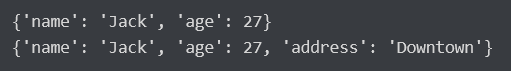##### Removing Elements from Dictionary in Python

Using the pop() method, we can remove a specific item from a dictionary. This method deletes an item with the specified key and returns its value. The popitem() method is used to remove and return any (key, value) item pair from the dictionary. Using the clear() method, all items can be removed at once. The del keyword can also be used to remove individual items or the entire dictionary.

```# Removing elements from a dictionary

# create a dictionary
squares = {1: 1, 2: 4, 3: 9, 4: 16, 5: 25}

# Remove a particular item, returns its value
# Output: 16
print(squares.pop(4))

# Output: {1: 1, 2: 4, 3: 9, 5: 25}
print(squares)

# remove an arbitrary item, return (key,value)
# Output: (5, 25)
print(squares.popitem())

# Output: {1: 1, 2: 4, 3: 9}
print(squares)

# remove all items
squares.clear()

# Output: {}
print(squares)

# delete the dictionary itself
del squares

# Throws Error
print(squares)
```
###### Output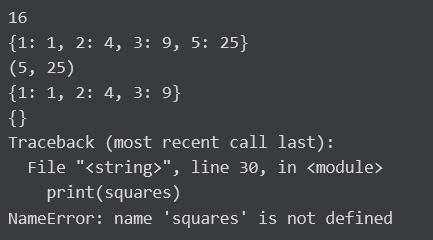#### Sets in Python:

A set is a collection of items that are not in any particular order. Every set element must be unique (no duplicates) and immutable (cannot be changed). A set, on the other hand, is mutable. We have the ability to add and remove items from it. Sets can also be used to perform mathematical set operations such as union, intersection, and symmetric difference, among others.

##### Creating Python Sets

A set is formed by enclosing all of the items (elements) within curly braces, separated by commas, or by using the built-in set() function. It can contain an unlimited number of items of various types (integer, float, tuple, string etc.). A set, on the other hand, cannot have mutable elements such as lists, sets, or dictionaries as its elements.

```# Different types of sets in Python
# set of integers
my_set = {1, 2, 3}
print(my_set)

# set of mixed datatypes
my_set = {1.0, "Hello", (1, 2, 3)}
print(my_set)
```
###### Output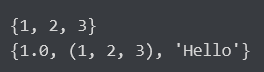##### Making a Set in Python

Sets can be changed. However, indexing has no meaning because they are not ordered. Indexing or slicing cannot be used to access or change a set element. It is not supported by the Set data type.

We can use the add() method to add a single element and the update() method to add multiple elements. The update() method can take as arguments tuples, lists, strings, or other sets. Duplicates are avoided in all cases.

```# initialize my_set
my_set = {1, 3}
print(my_set)

# my_set
# if you uncomment the above line
# you will get an error
# TypeError: 'set' object does not support indexing

# Output: {1, 2, 3}
print(my_set)

# Output: {1, 2, 3, 4}
my_set.update([2, 3, 4])
print(my_set)

# Output: {1, 2, 3, 4, 5, 6, 8}
my_set.update([4, 5], {1, 6, 8})
print(my_set)
```
###### Output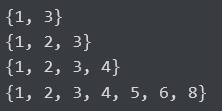##### Removing Elements from a Set in Python

The methods discard() and remove() can be used to remove a specific item from a set (). The only difference between the two is that the discard() function discards a set if the element is not present in it. The remove() function, on the other hand, will throw an error in such a case (if the element is not present in the set).

```# Difference between discard() and remove()

# initialize my_set
my_set = {1, 3, 4, 5, 6}
print(my_set)

# Output: {1, 3, 5, 6}
print(my_set)

# remove an element
# Output: {1, 3, 5}
my_set.remove(6)
print(my_set)

# not present in my_set
# Output: {1, 3, 5}
print(my_set)

# remove an element
# not present in my_set
# you will get an error.
# Output: KeyError

my_set.remove(2)
```
###### Output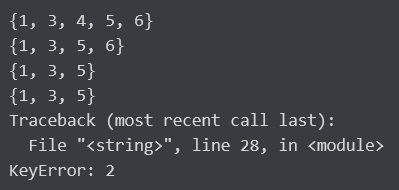In the next article, I am going to discuss Numpy and Pandas with Matplotlib and Seaborn for Data Science. Here, in this article, I try to explain Data Structures in Python with Examples. I hope you enjoy this Data Structures in Python with Examples article.